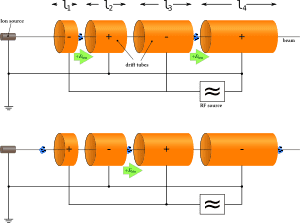# Finding the Electric Field of a Hollow Cylindrical Conductor

I am trying to find the electric field of a hollow, open-ended, thin-walled cylindrical conductor.

I am trying to solve something regarding LINACs:Assume we have an isolated, hollow, open-ended, thin-walled cylindrical conductor, with a net charge.

The net electric field within the cylinder is ostensibly zero, but since it isn't a closed surface, is there a net electric field near the open boundaries of the cylinder?

I tried to solve it using integral methods, but I ended up having to integrate over a function involving elliptic integrals of the first kind, which is hard, and I'm stupid.## Answers and Replies

Gold Member
Cool problem. If you're interesting in a numerical solution why not do it numerically. Use some integration like trapezoidal integration over the charges.

hackhard
The net electric field within the cylinder is ostensibly zero
no it can never be zero for a finite hollow cylinder,(except on points lying on mid plane (normal to axis) of cylinder which i doubt may also be nonzero but at midpoint of axis)
suppose take a point P on axis of cylinder at a distance 't' from one base of cylinder (t < (h/2)) where h is height of cylinder
field at P due to portion of cylinder below P (ie of height 't') has some non zero projection along axis = E1
now take portion of cylinder above P, divide it into 2 parts , 1st part which is symmetric(about P) to portion of cylinder below P, rest part is 2nd part
field at P due to 1st part (ie of height 't') has some non zero projection along axis which cancels E1 (since vertical component of force is in opposite dir)
field at P due to 2nd part (ie of height 'h-2t') has some non zero projection along axis
so there is net non-zero vertical projection of field at any point on axis inside finite hollow cylinder (except at midpoint of axis)
so resultant field strength vector must be nonzero in magnitude for any point inside finite hollow cylinder
(
except on points lying on mid plane (normal to axis) of cylinder which i doubt may also be nonzero but at midpoint of axis)